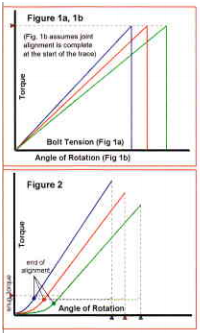# Torque Control vs. Angle Control

By | August 3, 2016

In previous columns. I’ve explained why tightening fasteners to a particular torque does not mean the resulting bolt tension will be either accurate or repeatable. That’s one of the biggest challenges of using bolted joints that depend on clamp load. However, another means of tightening that does not rely on the torque tension relationship is available to manufacturing engineers. This method is often called “angle control” in assembly and “turn-of-the-nut’ in construction applications. It is a more fundamentally sound method of tension control. There is always a direct correlation between bolt elongation and bolt tension until the bolt yields, which is not the case with the torque-tension relationship. Differences between torque and angle control are more easily understood visually. Figure la could be the result of a bench test used to calculate friction coefficient or nut factor, where the resulting tension can vary for a given torque input. The amount of variation depends on geometry, surface finish and coatings, but the extent in Figure I is not exaggerated for some conditions. Unfortunately, electroplated zinc, the most common fastener coating, has some of the worst torque-tension variation.

Assuming the trace occurs in the elastic range, angle can be substituted for tension on the X axis (Figure lb) to show how this condition impacts traditional torque-controlled tightening. Because the friction effect of each bolt varies, the angle the bolt rotates before the installation torque is reached is not constant. Higher friction will cause torque to rise more quickly. Because the angle of rotation is smaller, the bolt tension generated is also proportionally less. This is the theory behind angle control. Since torque only indirectly creates tension while the bolt elongation that results from rotation is directly proportional to it, let’s rotate to a specified angle rather than a specified torque and we’ll always get the desired tension. Of course, it’s not that simple in actual use.

First, we need to determine the angle that will achieve our desired tension. If we were elongating the bolt as we would a steel rod in a tensile tester, Hooke’s Law would make it easy to estimate the elongation required for a given tension. However, for every unit of bolt elongation in a joint, there is a corresponding (but unequal) amount of compression in the clamped components. For simple hard joints, such as non gasketed steel plates, this compression can be calculated by the same method used for bolt elongation. For other less ideal joints, testing is required in fact, testing is needed to utilize angle control for non ideal joints for another reason. Joint stiffness, represented by the slope of a tension-angle trace, is not constant while the joint is being drawn together into complete contact. This is represented in Figure 2, which also illustrates a common solution to the problem. Testing determines the torque at which the transition phase ends and the linear port ion of the trace begins. Allowing for panlo-part variation, this “snug” torque is determined. The specified angle of rotation, which must be measured with sufficient accuracy, starts from this point. As bolt length-to-diameter ratios get smaller, more tension is generated per degree of rotation, so greater accuracy is required.

Less common, but more troublesome, is when the tension-angle trace is not linear, due to joint stiffness variation. While testing is required to establish angle control on all but the simplest joints, this is also true of torque control where in-joint tests or verified friction factors are needed. So, the bottom line comparison between angle control and torque control is that joints with consistent stiffness and variable friction favor angle control, while consistent friction and variable stiffness favors torque control. Joints with neither consistent stiffness nor friction require a return to the drawing board. Since the first joint type is the most common, and torque control is definitely more common in assembly applications, there are opportunities for many manufacturers to successfully employ angle control, particularly those using tightening tools with angle encoders.Written by Dave Archer
Principal Engineer for Peak innovations Engineering